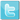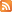# Api hash gen

Author: David Zimmer
Date: 01.09.11 - 5:45am

Its pretty typical for shellcode to use an API hasher to locate its target functions. There are some variations in the number of bits they shift to generate the hash. Below is a quick sample in C of a hasher stub where you can just pass in the string api name, and the number of bits to shift and it will output the hash.

I have also included it as a byte buffer VB6 developers can use with the help of CallWindowProc (and its development stub).

```
Sample Output:

/*
ror7.WinExec = 0x01A22F51
rorD.WinExec = 0x0E8AFE98
rorD.WinExec = 0x0E8AFE98 (CallWindowProc Example)
rorD.WinExec = 0x0E8AFE98 (CallWindowProc byte buffer example)
Brute forcing hash 0xE70283B6
Found bit shift used for WinExec known hash 0xE70283B6 = 0x13

rorD.CloseHandle = 0x0FFD97FB
ror7.CloseHandle = 0xFF0D6657
ror7.CloseHandle = 0xFF0D6657 (CallWindowProc Example)
ror7.CloseHandle = 0xFF0D6657 (CallWindowProc byte buffer example)
*/

#include
#include

char* vbUsable[] = {
"x8Bx45x0CxBBx00x00x00x00xB9x00x00x00x00x8A"
"x4Dx10x0FxBEx10x3AxD6x74x07xD3xCBx03xDAx40"
"xEBxF2x8BxC3xC2x10x00"
};

int _declspec(naked) hasher_4_CallWindowProc(int dummy, char* api, char bits, int arg4){

//printf("in myWindowProc api=%s bits=%x", api, bits);

_asm{

mov     eax, api   ;load our string pointer
mov     ebx, 0
mov     ecx, 0
mov     cl, bits

hash_loop:
movsx   edx, byte ptr [eax]  ;get next char
cmp     dl, dh               ;reached end of string?
jz      short end_of_string
ror     ebx, cl
inc     eax
jmp     short hash_loop

end_of_string:
mov eax, ebx
//mov retVal, eax

}

//printf("Final value is: %X", retVal);

_asm retn 10h

}

int hasher(char* api, char bits){

int retVal=0;

_asm{

mov     eax, api   ;load our string pointer
mov     ebx, 0
mov     ecx, 0
mov     cl, bits

hash_loop:
movsx   edx, byte ptr [eax]  ;get next char
cmp     dl, dh               ;reached end of string?
jz      short end_of_string
ror     ebx, cl
inc     eax
jmp     short hash_loop

end_of_string:
mov retVal, ebx

}

return retVal;

}

uint32_t __stdcall proto_CallWindowProc_rol32_generic(
int dummy, const uint32_t x , int i, int arg4)
{
return (x << static_cast(i & 31)) |
(x >> static_cast((32 - (i & 31)) & 31));

}

void main(void){

int  x = 0;
unsigned char bits;
char *api = "CloseHandle";
int ror13_test = 0xE70283B6;

//this is for using vc to generate the asm for use in vb6 w/CallWindowProc

bits = 0xD;
printf("ror%X.%s = 0x%08X
", bits, api, hasher(api, bits) );

bits = 0x7;
printf("ror%X.%s = 0x%08X
", bits, api, hasher(api, bits) );

_asm{
push 0
push 0
push bits
push api
push offset hasher_4_CallWindowProc
call lpfnCallWindowProc
mov  x, eax
}

printf("ror%X.%s = 0x%08X (CallWindowProc Example)
", bits,api, x);

_asm{
push 0
push 0
push bits
push api
push vbUsable
call lpfnCallWindowProc
mov  x, eax
}

printf("ror%X.%s = 0x%08X (CallWindowProc byte buffer example)
", bits,api, x);

printf("
Brute forcing hash 0x%X
", ror13_test);

for(bits=1;bits<254;bits++){
if(hasher("WinExec",bits) == ror13_test){
printf("Found bit shift used for WinExec known hash 0x%X = 0x%X
", ror13_test, bits);
exit(0);
}
}

printf("
Could not brute force hash");

}

Example VB6 code to call this byte buffer as asm:

Private Declare Function CallWindowProc Lib "user32"
Alias "CallWindowProcA" (
ByVal lpPrevWndFunc As Long,
ByVal hWnd As Long,
ByVal Msg As Long,
ByVal wParam As Long,
ByVal lParam As Long
) As Long

Const ror = "x8Bx45x0CxBBx00x00x00x00xB9x00x00x00x00x8A" & _
"x4Dx10x0FxBEx10x3AxD6x74x07xD3xCBx03xDAx40" & _
"xEBxF2x8BxC3xC2x10x00"

Const asm_rol32 = "x55x8BxECx56x8Bx4Dx10x83xE1x1Fx8Bx45x0C" & _
"xD3xE0x8Bx4Dx10x83xE1x1FxBAx20x00x00x00" & _
"x2BxD1x83xE2x1Fx8Bx75x0Cx8BxCAxD3xEEx0B" & _
"xC6x5Ex5DxC2x10x00"

Dim asm() As Byte
Dim asm2() As Byte
Const LANG_US = &H409

Function toBytes(s) As Byte()
Dim b() As Byte
tmp = Split(s, "x")
ReDim b(UBound(tmp))
For i = 1 To UBound(tmp)
If Len(tmp(i)) > 0 Then
b(i) = CByte(CInt("&h" & tmp(i)))
End If
Next
toBytes = b()
End Function

Function rol32(base As Long, bits As Integer)
rol32 = CallWindowProc(VarPtr(asm2(1)), 0, base, bits, 0)
End Function

Function GetHash(api As String, bits As Integer)
Dim asciiName() As Byte
asciiName() = StrConv(api & chr(0), vbFromUnicode, LANG_US)
GetHash = CallWindowProc(VarPtr(asm(1)), VarPtr(asciiName(0)), 7, 0, 0)
End Function

asm() = toBytes(ror)
asm2() = toBytes(asm_rol32)
MsgBox Hex(GetHash("WinExec", 7))
MsgBox Hex(rol32(1, 1))
End Sub

```

On 01.09.11 - 9:21am Dave wrote:
 In the vb code, the conversion to asciiName I forgot to append the chr(0) on the end for a while. Worked allot, failed allot. That was a shitty bug to find!

On 11.20.13 - 11:27pm Daniel wrote:
 I wonder if we could get the original string from a hash value.

On 11.21.13 - 5:17am Dave wrote:
 see the next api hashs redux post. Since all the strings are known API names, you can easily figure out what string its looking for by building a database of known hash/string pairs.

On 08.27.14 - 6:33am Jay wrote:
 @Daniel - Most definitely you can even without a rainbow table such as the one Dave suggested. Youre looking for collisions as a hash is an accumulation of prime multiplied predicates (though this example uses bit-shifting to accomplish it) and is therefore classifiable as a lossful compression. So, and its actually better to use something like CUDA (or whatever AMDs knock off of it is) to stream hundreds of permutations per cycle from different starting points equally distributed until a given set of characters has the equivalent hash value. For example, the commonly used CRC32 hash an equal value for both plumless and buckeroo. Daves example is easily optimized with SCASB and for Drivers (as they use 16bit unicode) SCASW. That said Ive seen some clever people altogether bypass an obvious hash lookup by using a packet payload and having the NIC calculate a check-sum instead due to the fact it is unilaterally consistent algorithm. More complex shellcodes actually create API databases with hash tables - the concept is to overcome API collisions by using the hashed module name, or in the event the name has been stripped, the hash of the module in its entirety. Alternatively a code check for a known function is also feasible by using ordinal exports only(useful when dealing with obfuscated code such as EasyAntiCheat and other malware masquerading as services).

 Leave Comment: Name: Email: (not shown) Message: (Required) Math Question: 88 + 38 = ? followed by the letter: JTwitterRSS